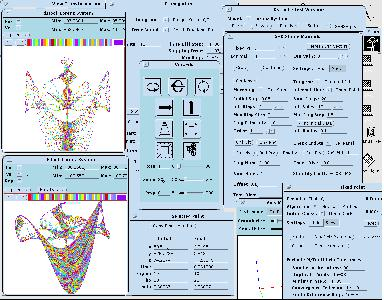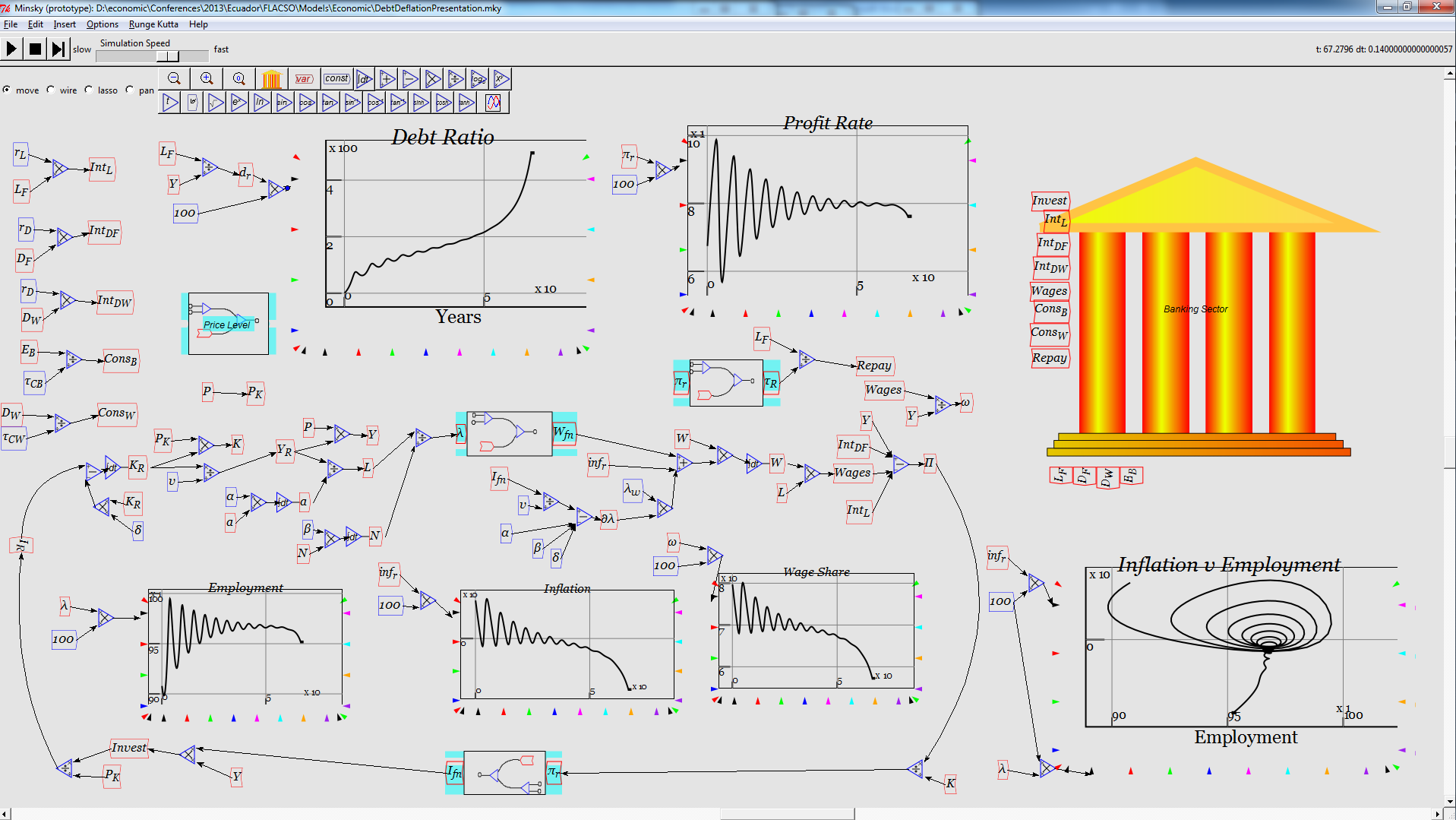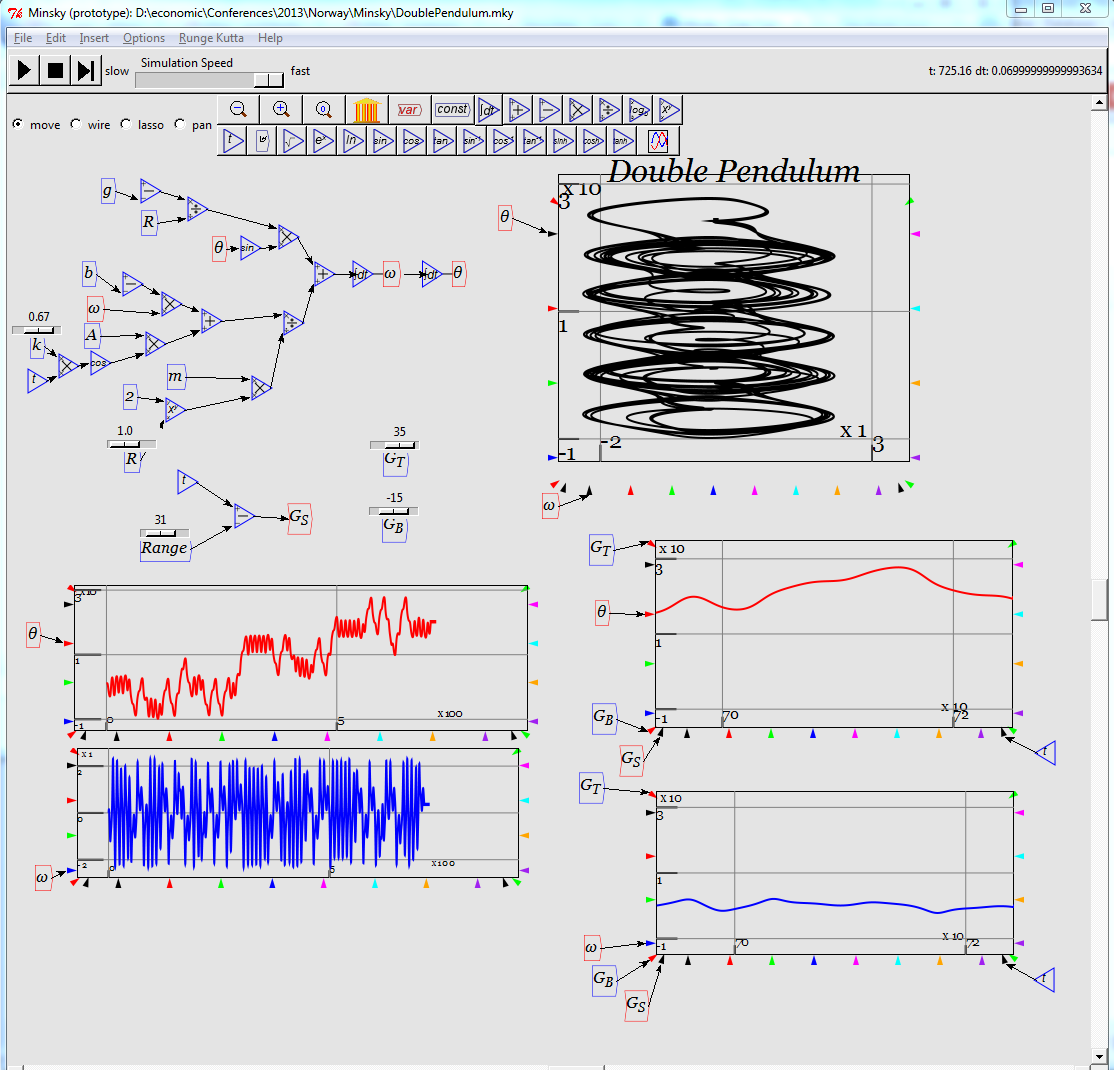## Ordinary Differential Equations

Or ODE's.

Express a function via its derivative. Often used to model physical systems mathematically.

For example radio-active decay proposes that the rate of decay is purely dependent on the number of atoms that have not yet decayed. So Rate of Change of Number = -constant*number.

or d/dt(Number) = -constant*number

The solution to this trivial (can such an important equation be trivial?) equation is

N=N0 * exp (-constant * time).

where N0 is the number of atoms at time = 0. (Just differentiate the function). If you havent studied calculus yet, dont worry perhaps this page is not for you yet.

If constant is negative then the number of atoms would grow. This situation can occur for living organisms - the number of new babies is proportional to the number of adults.

see Runge-Kutta for a method of solving ODE's numerically.

AM A number of the commonly used numerical methods can be found in Tcllib's math module.

Partial Differential Equations are much tougher to solve, particularly in 3 dimensions. Many solver programs exist, but few are suitable for Tcl (a fully compiled language is indicated).

tclode

DsTool is a program for interactively exporing the dynamics of dynamical systems. It allows you to draw trajectories, to find fixed points or bifurcation points. Dynamical systems arise in many disciplins of physics, biology and chemistry - basically always whenever something can be described by a set ordinary differential equations. The methods employed by the theory of dynamical systems allow to compute important properties directly without the need for long brute-force simulations.

Source code with Tk GUI can be downloaded from: [L1 ]See: Minsky : enables the simulation of models (particularly from economics) defined in terms of couple ordinary differential equations. The models are defined using a drawing canvas (rather like VisSim), and a double entry bookkeeping system known as a Godley table.

Screenshots:Category Mathematics